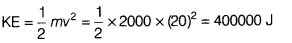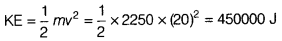If in a same bus 4 more passenger of total 250 kg sits, then find the new kinetic energy

1. If a bus of 2000 kg is moving with a velocity of 72 kmh–1, then calculate the kinetic energy.
2. If in a same bus 4 more passenger of total 250 kg sits, then find the new kinetic energy.
1. Given, m = 2000 kg, v = 80 kmh–1
Kinetic energy,2. Now the total mass, m = 2000 + 205 = 2250 kg
New ,